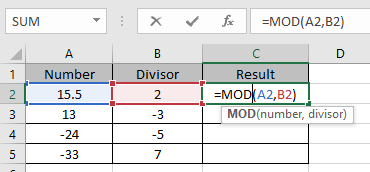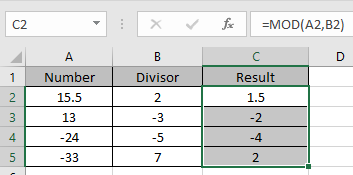# How to use the MOD Function in Excel

In this article, we will learn about how to use MOD function in Excel to calculate the remainder when the number is divided by the divisor.

MOD function is a built-in function to calculate the remainder when the number is divided by the divisor. It always has the same sign as divisor no matter the sign of remainder.
Syntax:

=MOD (number, divisor)

Let’s understand this function using it an example.Here we have some numbers and their divisors
Use the formula to get the remainder using MOD function

=MOD(A2,B2)Applying the formula in the remaining cellsAs you can see the result for the remainder but the sign is same as the sign of divisor.
Hope you understood how to use MOD function in Excel. Explore more articles on Simple Mathematical Excel function here. Please feel free to state your query or feedback for the above article.

Related Articles:

Simple interest formula in Excel

Excel IPMT Function

Calculate Profit margin percentage

Excel CUMIPMT Function

How to Use RATE Function in Excel

Popular Articles:

How to use the VLOOKUP Function in Excel

How to use the COUNTIF function in Excel 2016

How to Use SUMIF Function in Excel

Terms and Conditions of use

The applications/code on this site are distributed as is and without warranties or liability. In no event shall the owner of the copyrights, or the authors of the applications/code be liable for any loss of profit, any problems or any damage resulting from the use or evaluation of the applications/code.